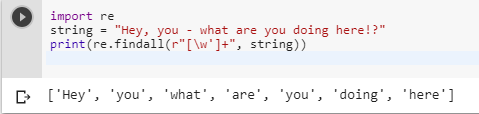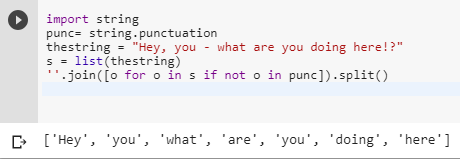2 views
in Python

I think what I want to do is a fairly common task but I've found no reference on the web.I have text with punctuation, and I want a list of the words.

"Hey, you - what are you doing here!?"

should be

['hey', 'you', 'what', 'are', 'you', 'doing', 'here']

But Python's str.split() only works with one argument, so I have all words with the punctuation after I split with whitespace. Any ideas?

+1 vote
by (106k points)
• There are many ways to solve this problem efficiently:-

The first way is you can use regular expression’s (A regular expression in a programming language is a special text string used for describing a search pattern.) method re .findall().

In a regular expression, we use two expressions that search pattern, those expressions are “^” and “w+”.

• "^": It matches the start of a string.

• "w+": It matches the alphanumeric character in the string.

re.findall():-

The re.findall() module is used when you want to iterate over the lines of the file, it will return a list of all the matches in a single step.

• Here’s an example of code that illustrates the above definition:-

import re

string = "Hey, you - what are you doing here!?"

print(re.findall(r"[\w']+", string))• Another way you can use to solve this problem is:-

We can use a string method to solve this problem which is as follows.

import string

punc= string.punctuation

thestring = "Hey, you - what are you doing here!?"

s = list(thestring)

''.join([o for o in s if not o in punc]).split()